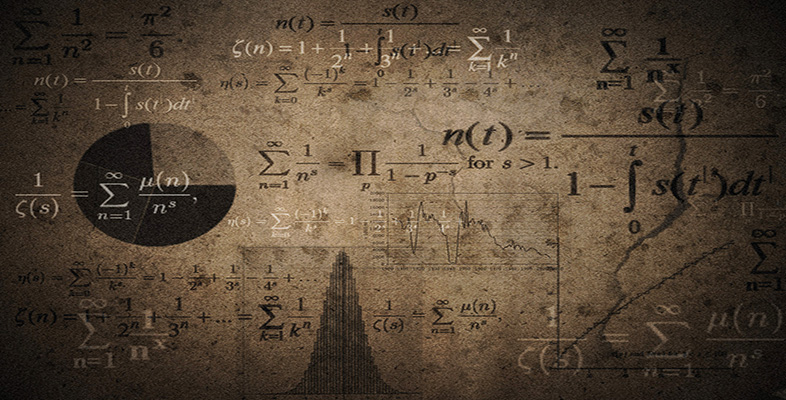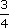Language, notation and formulas

Start this free course now. Just create an account and sign in. Enrol and complete the course for a free statement of participation or digital badge if available.

Free course

# 2.5.1 Try some yourself

## Activity 5

How would you add the following words to the list:

decimal, fraction, positive, negative.

For each one, give the mathematical meaning and an example of its use.

There are many different correct answers to this question. However, make sure that the meanings you have given are equivalent to the ones below.

WordMathematical meaningExample of use
DecimalA number expressed in terms of tenths, hundredths, etc.A quarter expressed as a decimal is 0.25.
FractionOne whole number over another.0.75 expressed as a fraction is.
PositiveGreater than zero.2 is a positive number.
NegativeLess than zero.2 is a negative number.
MU120_4M6